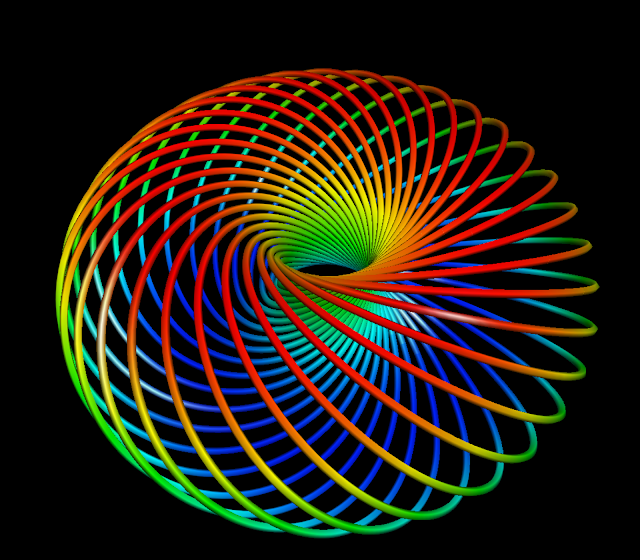## Friday, September 11, 2015

### Clifford Circles30 Clifford circles with with $\varphi = \pi/8$ and $\theta_0$ ranging from $0$ to $2\pi$
A discussion about Clifford tori would never be complete without a corresponding discussion about Clifford circles. These were featured as the logo of the UCSD math site for many years (not the case anymore, though, but I saved a screenshot!):

Just as the Clifford tori foliate $S^3$, the Clifford circles foliate each of the Clifford tori. As part of ongoing efforts to revisit algebraic topology, this example is one of the best to explore. We begin with classical mapping, called the Hopf fibration, which maps $S^3$ to $S^2$ by
$p\begin{pmatrix}x_1\\x_2\\x_3\\ x_4\end{pmatrix} =\begin{pmatrix}2(x_1 x_3 + x_2 x_4)\\ 2(x_2 x_3 -x_1x_4)\\ x_1^2 + x_2^2 -x_3^2 -x_4^2\end{pmatrix}.$
In fancy-schmancy homotopy-theory speak, $p$ is a generator of $\pi_3(S^2)$. Considering our previous parametrization $F$ from last time:
$F\begin{pmatrix}\varphi \\ \alpha \\ \beta\end{pmatrix} = \begin{pmatrix} \cos \alpha \cos \varphi \\ \sin\alpha\cos\varphi \\ \cos\beta\sin\varphi \\ \sin\beta\sin\varphi \end{pmatrix},$
we recall that the last coordinate of $p$ is simply $A^2 - B^2$, or $\cos^2(\varphi) - \sin^2(\varphi)$. Although I tell my students to not bother memorizing trigonometric identities, we derived that from a $\sin^2 \alpha + \cos^2 \alpha = 1$ and $\sin^2 \beta + \cos^2 \beta = 1$. We can further simplify that last coordinate to $\cos(2\varphi)$.

The key property that we want to demonstrate is that each point of $S^2$ corresponds to a whole circle in $S^3$ (for any $\boldsymbol \xi$ in $S^3$, the circle in question is the inverse image $p^{-1}(\boldsymbol\xi)$), and that each of these circles corresponding to distinct $\boldsymbol \xi$, though disjoint, are nevertheless linked together.

To do this, we visit the first two coordinates:
$2(x_1x_3 + x_2x_4) = 2(\cos\alpha \cos\varphi \cos\beta \sin\varphi+ \sin\alpha\cos\varphi \sin\beta\sin\varphi)$ $= 2\sin\varphi\cos\varphi(\cos\alpha\cos\beta +\sin\alpha\sin\beta) = \sin(2\varphi) \cos(\alpha-\beta),$ where the last equation is gotten either by trolling the back of a calculus book for some trig identities, or using complex numbers. Similarly,
$2(x_2x_3 - x_1x_4) = 2(\sin\alpha \cos\varphi \cos\beta \sin\varphi- \cos\alpha\cos\varphi \sin\beta\sin\varphi)$ $= 2\sin\varphi\cos\varphi(\sin\alpha\cos\beta -\cos\alpha\sin\beta) = \sin(2\varphi) \sin(\alpha-\beta).$
All together, we have $p \circ F\begin{pmatrix}\alpha\\ \beta\\ \varphi\end{pmatrix} = \begin{pmatrix}\sin(2\varphi)\sin(\alpha-\beta) \\ \sin(2\varphi)\cos(\alpha-\beta) \\ \cos(2\varphi)\end{pmatrix}.$
Letting $\theta = \alpha-\beta$, we see that this looks almost like our standard parametrization of a $3$-sphere, except that the polar angle $\varphi$ is off by a factor of $2$. No matter, it's still a sphere; we just have to take care to remember that the range of this $\varphi$ is $[0,\pi/2]$ rather than $[0,\pi]$. This confirms, incidentally, that $p$ really maps onto the sphere, rather than merely mapping into some amorphous blob in $\mathbb{R}^3$, as one can only assume at first, because the destination of the map $p$ has $3$ coordinates. The important thing to realize is that given any $\boldsymbol \xi$ in $S^2$, there is a unique $\varphi_0$ in $[0,\pi/2]$ and $\theta_0$ in $[0,2\pi]$ that correspond, under the usual parametrization, to $\boldsymbol \xi$. So, to calculate $p^{-1}(\boldsymbol \xi)$, we have to see how much we can change $\alpha$, $\beta$, and $\varphi$ in order to always get $(\varphi_0,\theta_0)$. By our above computations, this is easy: $\alpha$ and $\beta$ must satisfy $\alpha - \beta = \theta_0$, and $\varphi = \varphi_0$ is already completely determined. So our only degree of freedom here is $\alpha-\beta$: given some point in the fiber $p^{-1}(\boldsymbol \xi)$, if we add the same thing to both $\alpha$ and $\beta$, we will stay in the fiber. This means the fiber has a parametrization that looks like
$\beta \mapsto F\begin{pmatrix}\theta_0 + \beta \\ \beta \\ \varphi_0\end{pmatrix} = \begin{pmatrix} \cos (\theta_0 + \beta) \cos \varphi \\ \sin(\theta_0 + \beta)\cos\varphi \\ \cos\beta\sin\varphi \\ \sin\beta\sin\varphi \end{pmatrix}.$
We finally finish things off by composing with the stereographic projection $P$ as before:
$\beta \mapsto \frac{1}{1-\sin\beta\sin\varphi } \begin{pmatrix}\cos (\theta_0 + \beta) \cos \varphi \\ \sin(\theta_0 + \beta)\cos\varphi \\ \cos\beta\sin\varphi \end{pmatrix}.$
Plotting this out, this gives us the nice circles shown at the start of the post. We'll continue to explore the properties of $p$ and its visualizations as we move along in algebraic topology.